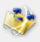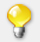# Tính toán thủy lựcCylinder Pressure (PSI) = Force (pounds)/Area (sq. Inch) PSI = F/A Cylinder Area (sq. inch) = 3.1416 x Radius Squared A = 3.1416 x R(sq.) Cylinder Force (pounds) = Pressure (PSI) x Area (sq. inch) F = P x A Cylinder Speed (ft./sec.) V = (231 x gpm) / (12 x 60 x Area) V = (0.3208 x GPM) / A Cylinder Volume, Gallons (Vol) = (Area x Stroke in inches) / 231 Vol. = (A x Stroke) / 231 Fluid Motor Torque (in. lbs.) T = Pressure (psi) x disp. (cu. in. / rev.) / 6.2822 T = (PSI x disp.) / 6.2822 Fluid Motor Speed (RPM) = (231 x GPM) / disp. (cu. in.) RPM = (231 x GPM) / disp. Fluid Motor Horsepower = Torque (in. lbs.) x RPM / 63025 HP = T x RPM / 63025 Pump Output Flow (GPM) = ((RPM) x disp. (cu. in.)) / 231 GPM = RPM / disp./231 Pump Input Horsepower = GPM x Pressure (psi) / 1714 x eff. HP = (Q x P) / 1714 x E Pump Input Torque (T) = (GPM x PSI x 3.06) / RPM /eff T = (GPM x PSI x 3.06) / RPM / eff Pump Displacement Cu. In. (Disp.) = GPM x 231) / RPM /eff Disp. = (GPM x 231) / RPM / eff Pump Input Speed ( RPM) = (GPM x 231) / Disp. / eff RPM = (GPM x 231) / Disp. / eff Pump Efficiency (eff) = Actual Flow (GPM) / Theoretical Flow (GPM) eff = Actual GPM / Ther GPM Pump Flow with PTO Drive (GPM) = (Engine RPM x % PTO x Disp. x eff) / 231 GPM = (Eng. RPM x % PTO x Disp. x eff) / 231 Velocity of Oil Flow in Pipe (Vel) = GPM x 0.3208 / Area Vel = (GPM x 0.3208) / Area Recommended Maximum Oil Velocity in Hydraulic Lines, FPS = Feet per Second Pump Suction: 2-4 FPS Pressure Lines to 3000 PSI: 15-20 FPS Pressure Lines over 3000 PSI: 25 FPS

"... còn rất nhiều sản phẩm, Quý Khách vui lòng liên hệ để chúng tôi gởi mẫu tham khảo."

SP bán chạy

 Bơm thủy lực cánh gạt có lưu lượng cố định
 Ty ren - Guzong, Ty giằng xà gồ
 Bu long neo (Bu long móng)
 Bu long cấp độ cao 8.8
 Bu long Inox SUS 201, SUS 304, SUS 316

Thành Viên

 Email Password Đăng ký   Quên mật khẩu?

Thống kêTổng số lượt truy cập

3,174,169Số người đang online

23